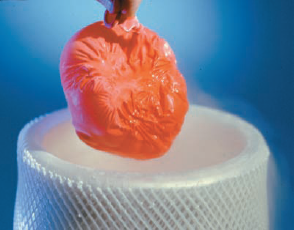×
Log in to StudySoup
Get Full Access to Introductory Chemistry - 5 Edition - Chapter 11 - Problem 127p
Join StudySoup for FREE
Get Full Access to Introductory Chemistry - 5 Edition - Chapter 11 - Problem 127p

Already have an account? Login here
×
Reset your password

# Solved: In a common classroom demonstration, a balloon is filled with air and submergedISBN: 9780321910295 34

## Solution for problem 127P Chapter 11

Introductory Chemistry | 5th Edition

• Textbook Solutions
• 2901 Step-by-step solutions solved by professors and subject experts
• Get 24/7 help from StudySoup virtual teaching assistantsIntroductory Chemistry | 5th Edition

4 5 1 294 Reviews
25
4
Problem 127P

PROBLEM 127P

In a common classroom demonstration, a balloon is filled with air and submerged into liquid nitrogen. The balloon contracts as the gases within the balloon cool. Suppose the balloon initially contains 2.95 L of air at 25.0 °C and a pressure of 0.998 atm. Calculate the expected volume of the balloon upon cooling to —196 °C (the boiling point of liquid nitrogen). When the demonstration is carried out, the actual volume of the balloon decreases to 0.61 L. How well does the observed volume of the balloon compare to your calculated value? Can you explain the difference?Step-by-Step Solution:
Step 1 of 3

Solution 127P

This has to do with Charles Law that states volume is proportional to temperature (if one goes up the other one does and vice versa).

The formula you must use is

v1/t1 = v2/t2

* v = volume

* t = temperature

Now substitute in the values... so...

2.94 L / 296 K = v2 / 77 K

v2 = (2.94 L / 296 K )(77 K)

v2 = 0.76 L

Observed volume of the balloon is the actual volume that occurred. Calculated volume of the balloon is what was expected based on math. Often these are not the same because little errors in experimental design.

Step 2 of 3

Step 3 of 3

##### ISBN: 9780321910295

Unlock Textbook Solution

Enter your email below to unlock your verified solution to:

Solved: In a common classroom demonstration, a balloon is filled with air and submerged